Gradient descent with Python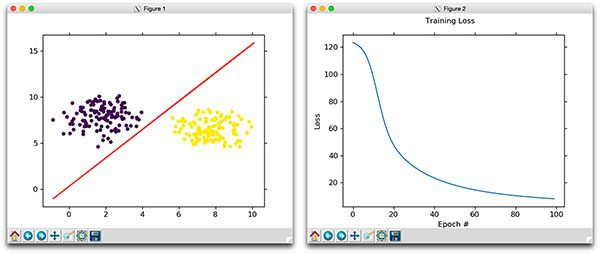Every relationship has its building blocks. Love. Trust. Mutual respect.

Yesterday, I asked my girlfriend of 7.5 years to marry me. She said yes.

It was quite literally the happiest day of my life. I feel like the luckiest guy in the world, not only because I have her, but also because this incredible PyImageSearch community has been so supportive over the past 3 years. Thank you for being on this journey with me.

And just like love and marriage have a set of building blocks, so do machine learning and neural network classifiers.

Over the past few weeks we opened our discussion of machine learning and neural networks with an introduction to linear classification that discussed the concept of parameterized learning, and how this type of learning enables us to define a scoring function that maps our input data to output class labels.

This scoring function is defined in terms of parameters; specifically, our weight matrix W and our bias vector b. Our scoring function accepts these parameters as inputs and returns a predicted class label for each input data point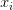$x_{i}$.

From there, we discussed two common loss functions: Multi-class SVM loss and cross-entropy loss (commonly referred to in the same breath as “Softmax classifiers”). Loss functions, at the most basic level, are used to quantify how “good” or “bad” a given predictor (i.e., a set of parameters) are at classifying the input data points in our dataset.

Given these building blocks, we can now move on to arguably the most important aspect of machine learning, neural networks, and deep learning — optimization.

Throughout this discussion we’ve learned that high classification accuracy is dependent on finding a set of weights W such that our data points are correctly classified. Given W, can compute our output class labels via our scoring function. And finally, we can determine how good/poor our classifications are given some W via our loss function.

But how do we go about finding and obtaining a weight matrix W that obtains high classification accuracy?

Do we randomly initialize W, evaluate, and repeat over and over again, hoping that at some point we land on a W that obtains reasonable classification accuracy?

Well we could — and it some cases that might work just fine.

But in most situations, we instead need to define an optimization algorithm that allows us to iteratively improve our weight matrix W.

In today’s blog post, we’ll be looking at arguably the most common algorithm used to find optimal values of W — gradient descent.

Looking for the source code to this post?

Gradient descent with Python

The gradient descent algorithm comes in two flavors:

1. The standard “vanilla” implementation.
2. The optimized “stochastic” version that is more commonly used.

Today well be reviewing the basic vanilla implementation to form a baseline for our understanding. Then next week I’ll be discussing the stochastic version of gradient descent.

Gradient descent is an optimization algorithm

The gradient descent method is an iterative optimization algorithm that operates over a loss landscape.

We can visualize our loss landscape as a bowl, similar to the one you may eat cereal or soup out of:Figure 1: A plot of our loss landscape. We typically see this landscape depicted as a “bowl”. Our goal is to move towards the basin of this bowl where this is minimal loss.

The surface of our bowl is called our loss landscape, which is essentially a plot of our loss function.

The difference between our loss landscape and your cereal bowl is that your cereal bowl only exists in three dimensions, while your loss landscape exists in many dimensions, perhaps tens, hundreds, or even thousands of dimensions.

Each position along the surface of the bowl corresponds to a particular loss value given our set of parameters, W (weight matrix) and b (bias vector).

Our goal is to try different values of W and b, evaluate their loss, and then take a step towards more optimal values that will (ideally) have lower loss.

Iteratively repeating this process will allow us to navigate our loss landscape, following the gradient of the loss function (the bowl), and find a set of parameters that have minimum loss and high classification accuracy.

To make our explanation of gradient descent a little more intuitive, let’s pretend that we have a robot — let’s name him Chad:Figure 2: Introducing our robot, Chad, who will help us understand the concept of gradient descent.

We place Chad on a random position in our bowl (i.e., the loss landscape):Figure 3: Chad is placed on a random position on the loss landscape. However, Chad has only one sensor — the loss value at the exact position he is standing at. Using this sensor (and this sensor alone), how is he going to get to the bottom of the basin?

It’s now Chad’s job to navigate to the bottom of the basin (where there is minimum loss).

Seems easy enough, right? All Chad has to do is orient himself such that he’s facing “downhill” and then ride the slope until he reaches the bottom of the basin.

But we have a problem: Chad isn’t a very smart robot.

Chad only has one sensor — this sensor allows him to take his weight matrix W and compute a loss function L.

Therefore, Chad is able to compute his (relative) position on the loss landscape, but he has absolutely no idea in which direction he should take a step to move himself closer to the bottom of the basin.

What is Chad to do?

The answer is to apply gradient descent.

All we need to do is follow the slope of the gradient W. We can compute the gradient of W across all dimensions using the following equation: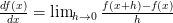$\frac{df(x)}{dx} = \lim_{h\to 0} \frac{f(x + h) - f(x)}{h}$

In > 1 dimensions, our gradient becomes a vector of partial derivatives.

The problem with this equation is that:

1. It’s an approximation to the gradient.
2. It’s very slow.

In practice, we use the analytic gradient instead. This method is exact, fast, but extremely challenging to implement due to partial derivatives and multivariable calculus. You can read more about the numeric and analytic gradients here.

For the sake of this discussion, simply try to internalize what gradient descent is doing: attempting to optimize our parameters for low loss and high classification accuracy.

Pseudocode for gradient descent

Below I have included some Python-like pseudocode of the standard, vanilla gradient descent algorithm, inspired by the CS231n slides:

This pseudocode is essentially what all variations of gradient descent are built off of.

We start off on Line 1 by looping until some condition is met. Normally this condition is either:

1. A specified number of epochs has passed (meaning our learning algorithm has “seen” each of the training data points N times).
2. Our loss has become sufficiently low or training accuracy satisfactorily high.
3. Loss has not improved in M subsequent epochs.

Line 2 then calls a function named evaluate_gradient . This function requires three parameters:

• loss : A function used to compute the loss over our current parameters W and input data .
• data : Our training data where each training sample is represented by a feature vector.
• W : This is actually our weight matrix that we are optimizing over. Our goal is to apply gradient descent to find a W that yields minimal loss.

The evaluate_gradient  function returns a vector that is K-dimensional, where K is the number of dimensions in our feature vector. The Wgradient  variable is actually our gradient, where we have a gradient entry for each dimension.

We then apply the actual gradient descent on Line 3.

We multiply our Wgradient  by alpha , which is our learning rate. The learning rate controls the size of our step.

In practice, you’ll spend a lot of time finding an optimal learning rate alpha  — it is by far the most important parameter in your model.

If alpha  is too large, we’ll end up spending all our time bouncing around our loss landscape and never actually “descending” to the bottom of our basin (unless our random bouncing takes us there by pure luck).

Conversely, if alpha  is too small, then it will take many (perhaps prohibitively many) iterations to reach the bottom of the basin.

Because of this, alpha  will cause you many headaches — and you’ll spend a considerable amount of your time trying to find an optimal value for your classifier and dataset.

Implementing gradient descent with Python

Now that we know the basics of gradient descent, let’s implement gradient descent in Python and use it to classify some data.

Open up a new file, name it gradient_descent.py , and insert the following code:

Lines 2-5 import our required Python packages.

We then define the sigmoid_activation  function on Line 7. When plotted, this function will resemble an “S”-shaped curve: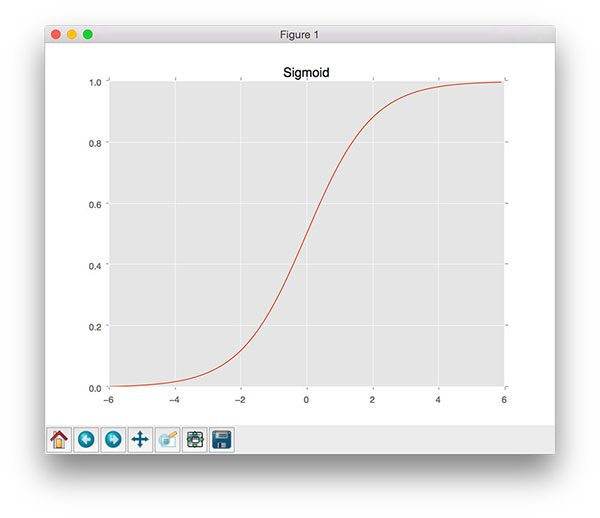Figure 4: A plot of the sigmoid activation function. Notice how y=0.5 when x=0.

We call this an activation function because the function will “activate” and fire “ON” (output value >= 0.5) or “OFF” (output vale < 0.5) based on the inputs x .

While there are other (better) alternatives to the sigmoid activation function, it makes for an excellent “starting point” in our discussion of machine learning, neural networks, and deep learning.

I’ll also be discussing activation functions in more detail in a future blog post, so for the time being, simply keep in mind that this is a non-linear activation function that we can use to “threshold” our predictions.

Next, let’s parse our command line arguments:

We can provide two (optional) command line arguments to our script:

• --epochs : The number of epochs that we’ll use when training our classifier using gradient descent.
• --alpha : The learning rate for gradient descent. We typically see 0.10.01, and 0.001 as initial learning rate values, but again, you’ll want to tune this hyperparameter for your own classification problems.

Now that our command line arguments are parsed, let’s generate some data to classify:

On Line 22 we make a call to make_blobs  which generates 250 data points. These data points are 2D, implying that the “feature vectors” are of length 2.

Furthermore, 125 of these data points belong to class 0 and the other 125 to class 1. Our goal is to train a classifier that correctly predicts each data point as being class 0 or class 1.

Line 29 applies a neat little trick that allows us to skip explicitly keeping track of our bias vector b. To accomplish this, we insert a brand new column of 1’s as the first entry in our feature vector. This addition of a column containing a constant value across all feature vectors allows us to treat our bias as a trainable parameter that is within the weight matrix W rather than an entirely separate variable. You can learn more about this trick here and here.

Line 34 (randomly) initializes our weight matrix such that it has the same number of dimensions as our input features.

It’s also common to see both zero and one weight initialization, but I tend to prefer random initialization better. Weight initialization methods will be discussed in further detail inside future neural network and deep learning blog posts.

Finally, Line 37 initializes a list to keep track of our loss after each epoch. At the end of our Python script, we’ll plot the loss which should ideally decrease over time.

All of our variables are now initialized, so we can move on to the actual training and gradient descent procedure:

On Line 40 we start looping over the supplied number of --epochs . By default, we’ll allow our training procedure to “see” each of the training points a total of 100 times (thus, 100 epochs).

Line 45 takes the dot product between our entire training data X  and our weight matrix W . We take the output of this dot product and feed the values through the sigmoid activation function, giving us our predictions.

Given our predictions, the next step is to determine the “error” of the predictions, or more simply, the difference between our predictions and the true values (Line 50).

Line 55 computes the least squares error over our predictions (our loss value). The goal of this training procedure is thus to minimize the least squares error.

Now that we have our error , we can compute the gradient  and then use it to update our weight matrix W :

Line 62 handles computing the actual gradient, which is the dot product between our data points X  and the error .

Line 68 is the most critical step in our algorithm and where the actual gradient descent takes place. Here we update our weight matrix W  by taking a --step  in the negative direction of the gradient, thereby allowing us to move towards the bottom of the basin of the loss landscape (hence the term, gradient descent).

After updating our weight matrix, we keep looping until the desired number of epochs has been met — gradient descent is thus an iterative algorithm.

To actually demonstrate how we can use our weight matrix W as a classifier, take a look at the following code block:

We start on on Line 72 by looping over a sample of our training data.

For each training point X[i]  we compute the dot product between X[i]  and the weight matrix W , then feed the value through our activation function.

On Line 81, we compute the actual output class label. If the activation  is < 0.5, then the output is class 0; otherwise, the output is class 1.

Our last code block is used to plot our training data along with the decision boundary that is used to determine if a given data point is class 0 or class 1:

To test our gradient descent classifier, be sure to download the source code using the “Downloads” section at the bottom of this tutorial.

From there, execute the following command:

Examining the output, you’ll notice that our classifier runs for a total of 100 epochs with the loss decreasing and classification accuracy increasing after each epoch: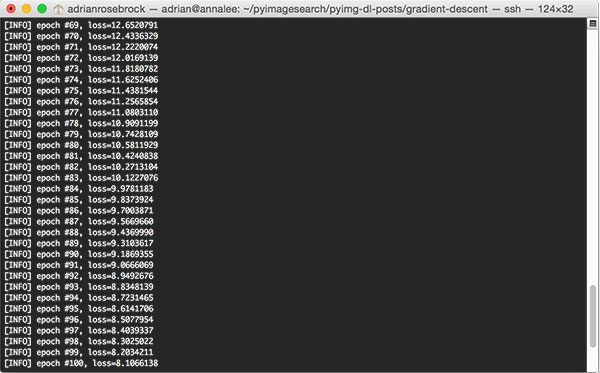Figure 5: When applying gradient descent, our loss decreases and classification accuracy increases after each epoch.

To visualize this better, take a look at the plot below which demonstrates how our loss over time has decreased dramatically: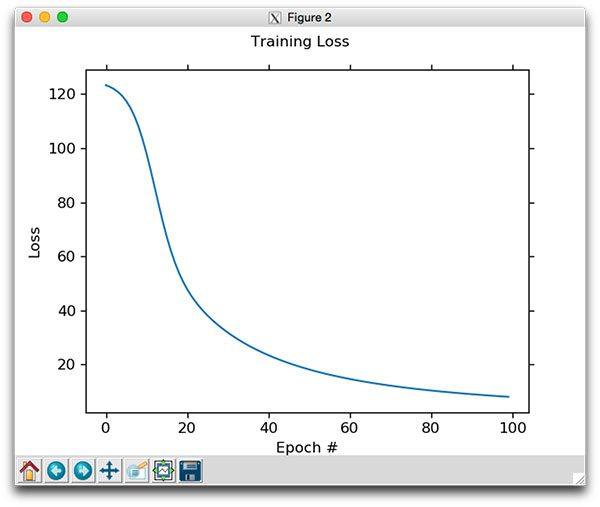Figure 6: Plotting loss over time using gradient descent. Notice how loss sharply drops and then levels out towards later epochs.

We can then see a plot of our training data points along with the decision boundary learned by our gradient descent classifier: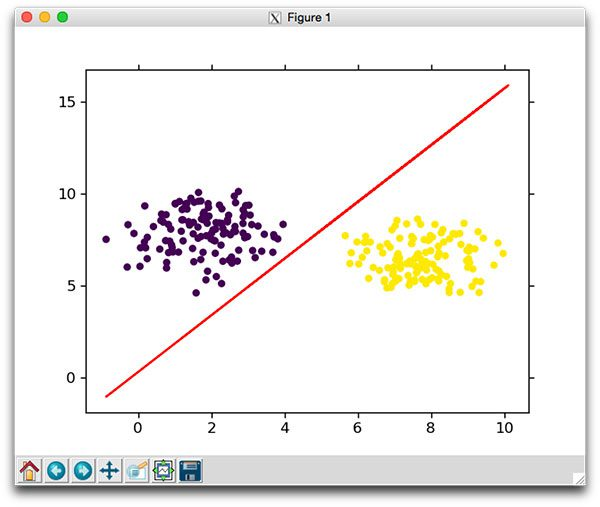Figure 7: Plotting the decision boundary learned by our gradient descent classifier.

Notice how the decision boundary learned by our gradient descent classifier neatly divides data points of the two classes.

We then then manually investigate the classifications made by our gradient descent model. In each case, we are able to correctly predict the class: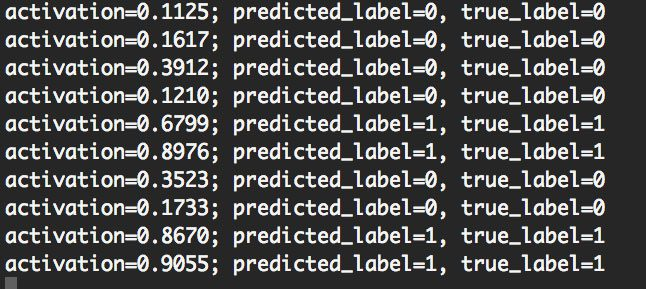Figure 8: Making predictions using our gradient descent classifier.

To visualize and demonstrate gradient descent in action, I have created the following animation which shows the decision boundary being “learned” after each epoch: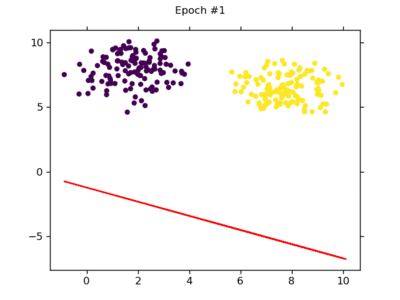Figure 8: An animation depicting how the decision boundary is learned via gradient descent.

As you can see, our decision boundary starts off widely inaccurate due to the random initialization. But as time passes, we are able to apply gradient descent, update our weight matrix W, and eventually learn an accurate model.

In next week’s blog post, I’ll be discussing a slight modification to gradient descent called Stochastic Gradient Descent (SGD).

In the meantime, if you want to learn more about gradient descent, you should absolutely refer to Andrew Ng’s gradient descent lesson in the Coursera Machine Learning course.

I would also recommend Andrej Karpathy’s excellent slides from the CS231n course.

Summary

In this blog post we learned about gradient descent, a first-order optimization algorithm that can be used to learn a set of parameters that will (ideally) obtain low loss and high classification accuracy on a given problem.

I then demonstrated how to implement a basic gradient descent algorithm using Python. Using this implementation, we were able to actually visualize how gradient descent can be used to learn and optimize our weight matrix W.

In next week’s blog post, I’ll be discussing a modification to the vanilla gradient descent implementation called Stochastic Gradient Descent (SGD). The SGD flavor of gradient descent is more commonly used than the one we introduced today, but I’ll save a more thorough discussion for next week.

See you then!

Before you go, be sure to use the form below to sign up for the PyImageSearch Newsletter — you’ll then be notified when future blog posts are published.If you would like to download the code and images used in this post, please enter your email address in the form below. Not only will you get a .zip of the code, I’ll also send you a FREE 17-page Resource Guide on Computer Vision, OpenCV, and Deep Learning. Inside you'll find my hand-picked tutorials, books, courses, and libraries to help you master CV and DL! Sound good? If so, enter your email address and I’ll send you the code immediately!

40 Responses to Gradient descent with Python

1.joe minichino October 10, 2016 at 11:26 am #

Congratulations Adrian. And yes, the article is also very interesting.

•Adrian Rosebrock October 11, 2016 at 12:59 pm #

Thank you very much Joe!

2.Jason October 10, 2016 at 11:31 am #

Didn’t have time to read this but CONGRATULATIONS! I hope your experience of marriage is as fulfilling and wonderful as mine has been.

•Adrian Rosebrock October 11, 2016 at 12:59 pm #

Thank you Jason! 🙂

3.Sujit Pal October 10, 2016 at 11:40 am #

Nice article. Just wanted to point out a typo. In your formula for d(f(x))/dx it should be limit of h tends to 0 not infinity.

•Adrian Rosebrock October 11, 2016 at 12:59 pm #

Thank you for pointing this out Sujit. I have updated the post.

4.Max Kostka October 10, 2016 at 12:17 pm #

Thanks for the informative post and the link to the slides, and I can totally recommend the machine learning course by andrew ng at coursera, too. It’s a real good introduction to different machine learning techniques. The hands on approach with the homework is worth every minute spent on it.

5.Johnny October 10, 2016 at 12:44 pm #

Fascinating! I love gradient descent studies like this one. I love Adrian’s teaching style. And mostly, love that you shared the news with us! Congratulations you two, many blessings and prayers coming at you!

•Adrian Rosebrock October 11, 2016 at 12:56 pm #

Thank you Johnny! 🙂

6.Ranjeet Singh October 11, 2016 at 2:19 am #

Great tutorial
Kidnly put some Backpropagation tutorials too.

•Adrian Rosebrock October 11, 2016 at 12:52 pm #

I will certainly be doing backpropagation tutorials, likely 2-3 of them. Right now it looks like I should be able to publish them in November/December following my current schedule.

7.Wajih October 11, 2016 at 4:10 am #

This explanation is so beautiful, simple and elegant…

•Adrian Rosebrock October 11, 2016 at 12:51 pm #

Thank you Wajih!

8.abkul October 12, 2016 at 2:43 am #

Thanks for the crystal clear explanation of the topic.

Congratulations for quiting bachelors club.

•Adrian Rosebrock October 13, 2016 at 9:19 am #

Thank you Abkul!

9.Moeen October 13, 2016 at 5:13 pm #

Hey Adrian- Big Congrats. I wish you the best!

•Adrian Rosebrock October 15, 2016 at 9:57 am #

Thanks Moeen!

10.Arasch U Lagies October 14, 2016 at 1:20 am #

•Adrian Rosebrock October 15, 2016 at 9:56 am #

Thank you Arasch!

11.vantruong57 October 16, 2016 at 10:51 am #

•Adrian Rosebrock October 17, 2016 at 4:07 pm #

Thank you!

12.Srinivasan Babu October 21, 2016 at 9:46 am #

nice article.

Thanks lot

•Adrian Rosebrock October 23, 2016 at 10:19 am #

Thank you Srinivasan!

13.Abkul October 31, 2016 at 2:18 am #

kindly do tutorials on “feature selection” in relation to deep learning

•Adrian Rosebrock November 1, 2016 at 9:04 am #

I’ll certainly be doing more deep learning tutorials in the future. Be sure to keep an eye on the PyImageSearch blog.

14.zauron November 2, 2016 at 6:49 pm #

Nice article! It’s my fault but I don’t understand how you calculate the decision boundary:

Y = (-W – (W * X)) / W

Could you elaborate a little more or give me some reference?

•dpereira December 1, 2016 at 8:38 am #

Hi zauron,

you can think of it like this:

In order to draw the decision boundary, you need to draw only the points (x,y) which lie right over the boundary.

According to the sigmoid function, the boundary is the value 0.5. So, in order to obtain a 0.5, you need to provide a zero value as input to the sigmoid (That is, a zero value as output from the scoring function).

Thus, if the scoring function equals zero:

0 = w0 + w1*x + w2*y ==> y = (-w0 – w1*x)/w2

You can use any x’s coordinates you want, and you’ll get the proper y’s coordinates to draw the boundary

15.Chahrazad January 23, 2017 at 2:45 am #

hello, it is a bit confusiong to me how the gradient was computed :

gradient = X.T.dot(error) / X.shape ,

shouldn’t this computation be true if the loss function was derived using maximum likelihood estimation not the squared error ?

16.foobar April 12, 2017 at 5:42 am #

Great stuff, thanks!

“In the meantime, if you want to learn more about gradient descent, you should absolutely refer to Andrew Ng’s gradient descent lesson in the Coursera Machine Learning course.

I would also recommend Andrej Karpathy’s excellent slides from the CS231n course.”

•Adrian Rosebrock April 12, 2017 at 12:58 pm #

Thank you for letting me know! I have updated both links so they are now working.

17.Tri Dao June 14, 2017 at 1:42 am #

I think the gradient is for logistic loss, not the squared loss you’re using.

18.Niki July 13, 2017 at 5:06 pm #

Thank you, an interesting tutorial! I’m a little bit confused though. When calculating the gradient, we try to minimize the loss function, which means we need to take the derivative of the loss function. The loss function is the sum of the square of the errors, with error being defined as the actual label minus the predicted label. Here the predicted labels are calculated using Sigmoid function. This means the gradient will include the gradient of the Sigmoid function, but here I see the gradient of a linear predictor function.
Could you elaborate more on what has been done. Thank you!

19.Niki July 13, 2017 at 7:21 pm #

My bad! I got error for W in the gradient formula.

•Adrian Rosebrock July 14, 2017 at 7:24 am #

Congrats on figuring it out Niki, nice job 🙂

•Kiro September 20, 2017 at 4:33 am #

I think you are right. There is a mistake. Shouldn’t it be like that:

gradient = X.T.dot(error) * preds*(1-preds)

where preds*(1-preds) is the derivative of the sigmoid function?

Thanks!

20.Ben August 17, 2017 at 10:27 am #

Congratulations on your pending nuptial.

•Adrian Rosebrock August 17, 2017 at 10:31 am #

Thanks Ben!

21.Dipankar September 30, 2019 at 5:17 pm #

Instead of sigmoid who can I use RelU activation function here?

•Adrian Rosebrock October 3, 2019 at 12:32 pm #

Yes, you can.

1. - October 17, 2016

[…] last week’s blog post, we discussed gradient descent, a first-order optimization algorithm that can be used to learn a set of classifier coefficients […]